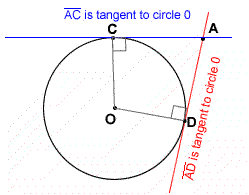Monday, September 14, 2009

Tangent Of A Circle.

I started the chapter on circle by introducing the concept of tangent to a circle.

A tangent to a circle has two defining properties

* A tangent touches a circle in exactly one point. This point is called point of tangency.
* The tangent intersects the circle's radius at a 90° angleWatch Video on Tangent Line

Properties of two tangents to a circleIf two tangents lines are drawn namely AP and BP, they will intersect at a point, P. The properties of the two tangent lines are listed below :

1. CA = CB
2. AP = BP
3. Angle ACP = Angle BCP
4. Angle Angle APC = Angle BPC
5. Angle CAP and angle CBP are both right angles
6. Triangle CAP and BCP are congruent